# Determining Safety Stock By Using Service Levels[Page 754 ( continued )]

There are several approaches to determining the amount of the safety stock needed. One of the most popular methods is to establish a safety stock that will meet a specified service level . The service level is the probability that the amount of inventory on hand during the lead time is sufficient to meet expected demand (i.e., the probability that a stockout will not occur). The word service is used because the higher the probability that inventory will be on hand, the more likely that customer demand will be met (i.e., the customer can be served ). For example, a service level of 90% means that there is a .90 probability that demand will be met during the lead time period and a .10 probability that a stockout will occur. The specification of the service level is typically a policy decision based on a number of factors, including costs for the "extra" safety stock and present and future lost sales if customer demand cannot be met.

The service level is the probability that the inventory available during the lead time will meet demand .

#### Reorder Point with Variable Demand

To compute the reorder point with a safety stock that will meet a specific service level, we will assume that the individual demands during each day of lead time are uncertain and independent and can be described by a normal probability distribution. The average demand for the lead-time period is the sum of the average daily demands for the days of the lead time, which is also the product of the average daily demand multiplied by the lead time. Likewise, the variance of the distribution is the sum of the daily variances for the number of days in the lead-time period. Using these parameters, the reorder point to meet a specific service level can be computed as follows :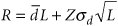where= average daily demand L = lead time s d = the standard deviation of daily demand Z = number of standard deviations corresponding to the service level probability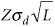= safety stock

[Page 755]

The term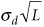in this formula for reorder point is the square root of the sum of the daily variances during lead time: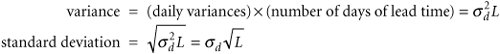The reorder point relative to the service level is shown in Figure 16.12. The service level is the shaded area, or probability, to the left of the reorder point, R .

##### Figure 16.12. Reorder point for a service level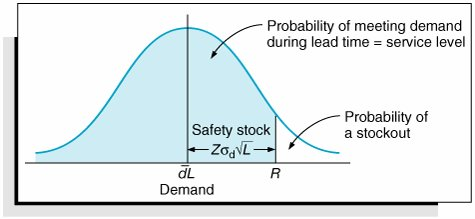The I-75 Carpet Discount Store sells Super Shag carpet. The average daily customer demand for the carpet stocked by the store is normally distributed, with a mean daily demand of 30 yards and a standard deviation of 5 yards of carpet per day. The lead time for receiving a new order of carpet is 10 days. The store wants a reorder point and safety stock for a service level of 95%, with the probability of a stockout equal to 5%:= 30 yd. per day, L = 10 days, s d = 5 yd. per day

For a 95% service level, the value of Z (from Table A.1 in Appendix A) is 1.65. The reorder point is computed as follows: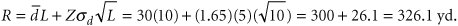The safety stock is the second term in the reorder point formula: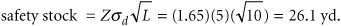#### Determining the Reorder Point Using Excel

Excel can be used to determine the reorder point with variable demand. Exhibit 16.6 shows an Excel spreadsheet set up to compute the reorder point for our I-75 Carpet Discount Store example. Notice that the formula for computing the reorder point in cell E7 is shown on the formula bar at the top of the spreadsheet.

[Page 756]
##### Exhibit 16.6.#### Reorder Point with Variable Lead Time

In the model in the previous section for determining the reorder point, we assumed a variable demand rate and a constant lead time. In the case where demand is constant and the lead time varies, we can use a similar formula, as follows:

R = d+ Zd s L

where

 d = constant daily demand= average lead time s L = standard deviation of lead time d s L = standard deviation of demand during lead time Zd s L = safety stock

For our previous example of the I-75 Carpet Discount Store, we will now assume that daily demand for Super Shag carpet is a constant 30 yards. Lead time is normally distributed, with a mean of 10 days and a standard deviation of 3 days. The reorder point and safety stock corresponding to a 95% service level are computed as follows:

 d = 30 yd. per day= 10 days s L = 3 days Z = 1.65 for a 95% service level R = d+ Zd s L = (30)(10) + (1.65)(30)(3) = 300 + 148.5 = 448.5 yd.

#### Reorder Point with Variable Demand and Lead Time

The final reorder point case we will consider is the case in which both demand and lead time are variables . The reorder point formula for this model is as follows.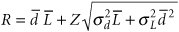where= average daily demand= average lead time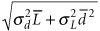= standard deviation of demand during lead time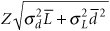= safety stock

[Page 757]

## Management Science Application: Establishing Inventory Safety Stocks at Kellogg's

Kellogg's is the world's largest cereal producer and a leading maker of convenience foods , with worldwide sales in 1999 of almost \$7 billion. The company started with a single product, Kellogg's Corn Flakes, in 1906, and over the years has developed a product line of other cereals, including Rice Krispies and Corn Pops, as well as convenience foods, such as Pop-Tarts and Nutri-Grain cereal bars. Kellogg's operates 5 plants in the United States and Canada and 7 distribution centers, and it contracts with 15 co-packers to produce or pack some Kellogg's products. Kellogg's must coordinate the production, packaging, inventory, and distribution of roughly 80 cereal products alone at these various facilities.

For more than a decade , Kellogg's has been using a model called the Kellogg Planning System (KPS) to plan its weekly production, inventory, and distribution decisions. The data used in the model are subject to much uncertainty, and the greatest uncertainty is in product demand. Demand in the first few weeks of a planning horizon is based on customer orders and is fairly accurate; however, demand in the third and fourth weeks may be significantly different from marketing forecasts. However, Kellogg's primary goal is to meet customer demand, and in order to achieve this goal, Kellogg's employs safety stocks as a buffer against uncertain demand. The safety stock for a product at a specific production facility in week t is the sum of demands for weeks t and t + 1. However, for a product that is being promoted in an advertising campaign, the safety stock is the sum of forecasted demand for a 4-week horizon or longer. KPS has saved Kellogg's many millions of dollars since the mid-1990s. The tactical version of KPS recently helped the company consolidate production capacity, with estimated projected savings of almost \$40 million.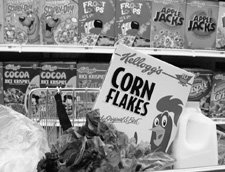Source: G. Brown, J. Keegan, B. Vigus, and K. Wood, "The Kellogg Company Optimizes Production, Inventory, and Distribution," Interfaces 31, no. 6 (NovemberDecember 2001): 115.

Again we will consider the I-75 Carpet Discount Store example, used previously. In this case, daily demand is normally distributed, with a mean of 30 yards and a standard deviation of 5 yards. Lead time is also assumed to be normally distributed, with a mean of 10 days and a standard deviation of 3 days. The reorder point and safety stock for a 95% service level are computed as follows: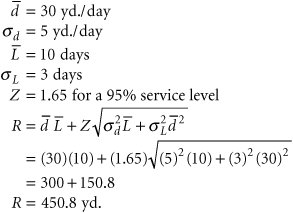[Page 758]

Thus, the reorder point is 450.8 yards, with a safety stock of 150.8 yards. Notice that this reorder point encompasses the largest safety stock of our three reorder point examples, which would be anticipated, given the increased variability resulting from variable demand and lead time.Introduction to Management Science (10th Edition)
ISBN: 0136064361
EAN: 2147483647
Year: 2006
Pages: 358

Similar book on Amazon

flylib.com © 2008-2017.
If you may any questions please contact us: flylib@qtcs.net